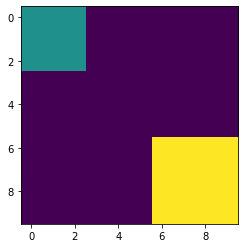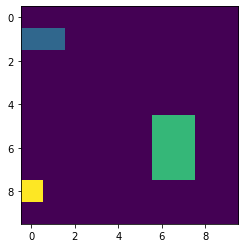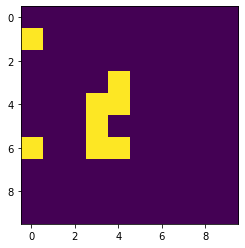# Mahotas – Edges using Difference of Gaussian for binary image

In this article we will see how we can edges of the binary image in mahotas with the help of DoG algorithm. In imaging science, difference of Gaussians (DoG) is a feature enhancement algorithm that involves the subtraction of one blurred version of an original image from another, less blurred version of the original.

In order to do this we will use `mahotas.dog` method

Syntax : mahotas.dog(img)

Argument : It takes binary image object as argument

Return : It returns image object

Below is the implementation

 `# importing required libraries ` `import` `mahotas as mh ` `import` `numpy as np ` `from` `pylab ``import` `imshow, show ` `   `  `# creating region ` `# numpy.ndarray ` `regions ``=` `np.zeros((``10``, ``10``), ``bool``) ` `   `  `# setting 1 value to the region ` `regions[:``3``, :``3``] ``=` `1` `regions[``6``:, ``6``:] ``=` `1` `   `  `# getting labeled function ` `labeled, nr_objects ``=` `mh.label(regions) ` `   `  `# showing the image with interpolation = 'nearest' ` `print``(``"Binary Image"``) ` `imshow(labeled, interpolation ``=``'nearest'``) ` `show() ` `  `  `# getting edges using dog algo ` `dog ``=` `mahotas.dog(labeled) ` `  `  `# showing image ` `print``(``"Edges using DoG algo"``) ` `imshow(dog) ` `show() `

Output :

`Binary Image``Edges using DoG algo`

Another example

 `# importing required libraries ` `import` `mahotas as mh ` `import` `numpy as np ` `from` `pylab ``import` `imshow, show ` `   `  `# creating region ` `# numpy.ndarray ` `regions ``=` `np.zeros((``10``, ``10``), ``bool``) ` `   `  `# setting 1 value to the region ` `regions[``1``, :``2``] ``=` `1` `regions[``5``:``8``, ``6``: ``8``] ``=` `1` `regions[``8``, ``0``] ``=` `1` `   `  `# getting labeled function ` `labeled, nr_objects ``=` `mh.label(regions) ` `   `  `# showing the image with interpolation = 'nearest' ` `print``(``"Image"``) ` `imshow(labeled, interpolation ``=``'nearest'``) ` `show() ` `  `  `# getting edges ` `dog ``=` `mahotas.dog(labeled) ` `  `  `# showing image ` `print``(``"Edges using DoG algo"``) ` `imshow(dog) ` `show() `

Output :

`Binary Image``Edges using DoG algo`My Personal Notes arrow_drop_upCheck out this Author's contributed articles.

If you like GeeksforGeeks and would like to contribute, you can also write an article using contribute.geeksforgeeks.org or mail your article to contribute@geeksforgeeks.org. See your article appearing on the GeeksforGeeks main page and help other Geeks.

Please Improve this article if you find anything incorrect by clicking on the "Improve Article" button below.

Article Tags :

Be the First to upvote.

Please write to us at contribute@geeksforgeeks.org to report any issue with the above content.